## Solution to 1986 Problem 45

 The word elastically" implies that both energy and momentum are conserved. Assume, the incoming photon is incident in the$x$ direction and scatters in the y direction. Then conservation of momentum gives: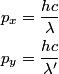\begin{align*}p_x &= \frac{h c}{\lambda} \\p_y &= \frac{h c}{\lambda'}\end{align*}where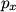$p_x$ and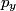$p_y$ are the x and y components of the protons momentum after the collision, and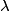$\lambda$ and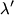$\lambda'$ are the photons wavelengths before and after the collision, respectively. The initial energy is: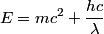\begin{align*}E = m c^2 + \frac{h c}{\lambda}\end{align*}The final energy is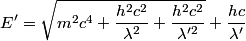\begin{align*}E' = \sqrt{m^2 c^4 + \frac{h^2 c^2}{\lambda^2} + \frac{h^2 c^2}{\lambda'^2}} + \frac{h c}{\lambda'}\end{align*}Equating the initial and final energy, we have that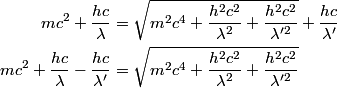\begin{align*}m c^2 + \frac{h c}{\lambda} &= \sqrt{m^2 c^4 + \frac{h^2 c^2}{\lambda^2} + \frac{h^2 c^2}{\lambda'^2}} + \f...Square both sides and solve for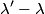$\lambda' - \lambda$ to find that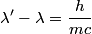\begin{align*}\lambda' - \lambda = \frac{h}{mc}\end{align*}So, answer (D) is correct.